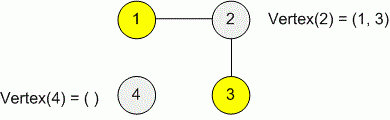bolt
Try our new interface for solving problems
Problems

# Operations on graph

Time limit 3 seconds
Memory limit 128 MiB

Create an undirected graph that supports the next operations:

1. AddEdge(u, v) - add to the graph an edge between the vertices (u, v);

2. Vertex(u) - print the list of vertices, adjacent with the vertex u.

There is no loops and multiple edges in the graph.

## Input data

The first line contains the number of vertices in a graph n (1n10^5). The next line contains the number of operations k (0k10^6). Each next line describes the operation in format: "1 " or "2 ", representing respectively the operation AddEdge(u, v) and Vertex(u).

It is guaranteed that the total amount of numbers to be printed during all operations Vertex, does not exceed 2 * 10^5.

## Output data

For each operation Vertex print in a separate line the list of adjacent vertices for a given vertex. The vertices in a list can be printed in any order. If some vertex hasn't adjacent vertices, print the empty line.## Examples

Input example #1
4
4
1 1 2
1 2 3
2 4
2 2

Output example #1
1 3